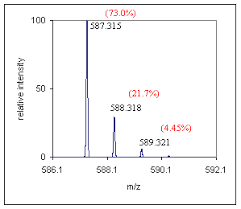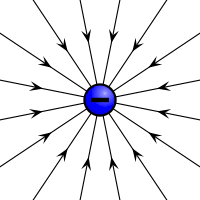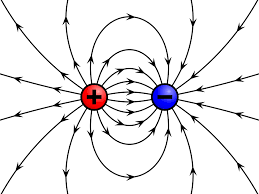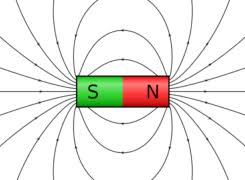## How to Calculate and Solve for the Quantity of Charge, Electrochemical Equivalence of a Substance and Mass of an Element in Electrolysis | Nickzom CalculatorThe image above represents the mass of an element.

To compute for the mass of an element, two essential parameters are needed and these parameters are Electrochemical Equivalence of the Substance (Z) and quantity of charge (Q).

The formula for calculating mass of an element:

M = ZQ

Where;
M = Mass of the element
Z = Electrochemical Equivalence of the Substance
Q = Quantity of Charge

Let’s solve an example;
Find the mass of an element when the Quantity of charge is 28 and Electrochemical Equivalence of the Substance is 32.

This implies that;
Z = Electrochemical Equivalence of the Substance = 32
Q = Quantity of Charge = 28

M = ZQ
M = 32 x 28
M = 896

Therefore, the mass of an element is 896 kg.

Calculating the Electrochemical Equivalence of the Substance when the Mass of an Element and Quantity of Charge is Given.

Z = M / Q

Where;
Z = Electrochemical Equivalence of the Substance
M = Mass of the element
Q = Quantity of Charge

Let’s solve an example;
Find the Electrochemical Equivalence of the Substance when the Quantity of charge is 12 and  mass of an element  is 120.

This implies that;
M = Mass of the element = 120
Q = Quantity of Charge = 12

Z = M / Q
Z = 120 / 12
Z = 10

Therefore, the Electrochemical Equivalence of the Substance is 10.

## How to Calculate and Solve for the Current, Time and Quantity of Charge of an Electrolysis | The Calculator EncyclopediaThe image above represents the quantity of charge.

To compute for the quantity of charge, two essential parameters are needed and these parameters are current (I) and time (T).

The formula for calculating the quantity of charge:

Q = It

Where;
Q = Quantity of charge
I = Current
T = Time

Let’s solve an example;
Find the quantity of charge with a current of 24 and time of 12.

This implies that;
I = Current = 24
T = Time = 12

Q = It
Q = 24 x 12
Q = 288

Therefore, the quantity of charge is 288 Coulombs (C).

Calculating the Current (I) using the Quantity of Charge and Time.

I = Q / t

Where;
I = Current
Q = Quantity of charge
T = Time

Let’s solve an example;
Given that the quantity of charge is 240 with a time of 14. Find the Current?

This implies that;
Q = Quantity of charge = 240
T = Time = 14

I = Q / t
I = 240 / 14
I = 17.14

Therefore, the current is 17.14 ampere.

## How to Calculate and Solve for the Current, Time and Quantity of Charge | Nickzom CalculatorThe image above represents the quantity of charge.

To compute the quantity of charge, two essential parameters are needed and the parameters are current (I) and time (t).

The formula for calculating the quantity of charge;

Q = It

Where;
Q = Quantity of Charge
I = Current
t = Time

Let’s solve an example;
Find the quantity of charge when the current (I) is 24 amp with a time of 8 secs.

This implies that;
I = Current = 24 amp
t = Time = 8 secs

Q = It
Q = 24 x 8
Q = 192

Therefore, the quantity of charge is 192 coulombs (C).

Calculating the Current (I) of a charge using the Quantity of Charge (Q) and the time (t).

I = Q / t

Where;
I = Current
Q = Quantity of the charge
t = Time

Let’s solve an example;
Find the current of a charge with the quantity of the charge as 150 coulombs (C) and time as 15 secs.

This implies that;
Q = Quantity of the charge = 150 C
t = Time = 15 secs

I = Q / t
I = 150 / 15
I = 10

Therefore. the current is 10 Ampere (A).

## How to Calculate and Solve for Magnetic Force | Nickzom CalculatorThe image above represents magnetic force.

To compute the magnetic force of a field, four essential parameters are needed and the parameters are Quantity of Charge (q), Average Velocity of the Charge (v), Magnetic Field Induction or Magnetic Flux Density (B) and Angle between v and B (θ).

The formula for calculating the magnetic force:

F = qVBsinθ

Where;
F = Magnetic Force
q = Quantity of Charge
v = Average Velocity of the Charge
B = Magnetic Field Induction or Magnetic Flux Density
θ = Angle between v and B

Let’s solve an example;
Find the magnetic force of a field when the Quantity of Charge (q) is 11, Average Velocity of the Charge (v) is 20, Magnetic Field Induction or Magnetic Flux Density (B) is 17 and Angle between v and B (θ) is 28°.

This implies that;
q = Quantity of Charge = 11
v = Average Velocity of the Charge = 20
B = Magnetic Field Induction or Magnetic Flux Density = 17
θ = Angle between v and B = 28°

F = qVBsinθ
F = 11 x 20 x 17 x sin28°
F = 11 x 20 x 17 x 0.469
F = 1755.82

Therefore, the magnetic force is 1755.82 Newton (N).